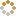﻿ N-B-U-T Online Judge ::  Hotline of Railway
• ###  Hotline of Railway

• 时间限制: 4000 ms　内存限制: 65535 K
• 问题描述
• The railway is finished. It opens up a hotline; you can query how many stone are there on a segment of the railway.

At the very beginning, all units have 0 stones.

There're 3 operations:

A n x y: Add n stones per unit from x to y.

D n x y: Reduce n stones per unit from x to y. If any unit is less then n stones, it will reduce to 0.

Q x y: The hotline received a query. You should tell the sum of stones from x to y.

(0 < n < 100, 0 <= x <= y <= 200000)

• 输入
• This problem has several cases.
The first line of each case is an integer N (0 < N < 10000), indicates how many operations.
Then follows N lines. Each line contains an operation. The rules are above.
• 输出
• For each query, print the sum. One answer per line.
• 样例输入
• ```2
A 1 1 100
Q 1 10
```
• 样例输出
• ```10
```
• 提示
• `无`
• 来源
• `XadillaX`
• 操作
﻿